Space, shape and measurement: Measure and calculate physical quantities

# Unit 1: Scales and measurement

Dylan Busa### Unit outcomes

By the end of this unit you will be able to:

• Use a ruler and protractor accurately.
• Understand the SI system of measurement.
• Convert between different units.

## What you should know

Before you start this unit, make sure you can:

• Do basic arithmetic calculations (adding, subtracting, dividing and multiplying) on a calculator.
• Use a ruler to measure lengths.
• Use a protractor to measure angles.

## Introduction

Imagine you go to the shops to buy some milk. When you get there all the bottles are different sizes and none of them show you how much milk is in them (figure 1).

How would you know how much milk you are getting and whether you are paying a fair price?

Thankfully, this is not how things work. When you go to the shops all the bottles of milk are labelled with how much they contain. We measure the amount of milk (and other liquids) by their volume in litres and millilitres.

We measure solids such as sugar in kilograms and grams (figure 2) and we measure things like fabric and rope in metres and centimetres.

When we measure something, we give a number to a characteristic of an object or event so that we can more accurately describe it and can compare it with other similar objects or events. Measurement is the cornerstone of trade, science and technology, and research. Think for a moment how much you depend on knowing the measurements of different things in your life.

## Systeme Internationale (SI) units

But how do you know that the litre of milk you buy from shop A is exactly the same amount as the litre of milk you buy at Shop B? How do you know that a $\scriptsize 1\ \text{kg}$ bag of sugar contains $\scriptsize 1\ \text{kg}$?

In order to make sure that a metre or a kilogram or a litre is always the same everywhere, we use an international system of measures and units called the Systeme Internationale (SI). This system reduces all physical measurements to a mathematical combination of seven base units. These are the kilogram (mass), metre (length), candela (brightness), second (time), ampere (electricity), kelvin (temperature) and mole (number of particles of a substance). Every other physical measure you can think of (even the old Imperial measures of miles, pounds, feet and gallons) are based on these seven base units.

### Note

If you want to learn more about each of these units, then visit the SI Units website.

### Did you know?

Units like the kilogram and metre used to be based on physical objects kept in Paris. The problem was that the mass and length of these objects changed over time and, if you ever wanted to check your length or mass against these, you had to go all the way to Paris!

First, in 1983, the metre was redefined based on an exact universal constant; the distance travelled by light in a vacuum in $\scriptsize \displaystyle \frac{1}{{299\text{ }792\text{ }458}}$ seconds.

More recently, the kilogram was redefined based on immutable (unchanging) physical constants. If you have an internet connection, watch the video called “How We’re Redefining the kg”  to see how and why it has been redefined.

How We’re Redefining the kg (Duration: 9.48)### Note

Each measure in the SI system has its own specific unit abbreviation.
Kilogram – kg
Metre – m
Second – s
Ampere – A
Kelvin – K
Mole – mol
Candela – cd

Note how some of them are lower case and others are upper case. Also, there should always be a space between the value of the measure and the unit, for example $\scriptsize 12\ \text{m}$, $\scriptsize 0.45\ \text{kg}$, $\scriptsize 1.875\ \text{A}$, and $\scriptsize 67.452\ \text{s}$.

## Taking measurements

One of the most common measurements in everyday life is the measure of length. We measure the length of many different things. Can you name some of these?

Because we need to measure the lengths of so many different things, it is not always practical to take measurements in metres. Imagine measuring the distance from the earth to the moon or the width of a pencil in metres. It could be done but might not be very accurate.

Fortunately, the SI system includes a set of standard prefixes that allow us to expand the range of all the measurements, for example kilometres and millimetres. The prefix ‘kilo’ means $\scriptsize 1\ 000$ and the ‘milli’ means $\scriptsize 1\ 000\text{th}$. So $\scriptsize 1\ \text{km = }1\ 000\ \text{m}$ and $\scriptsize 1\ \text{mm }=\text{ }\displaystyle \frac{1}{{1\ 000}}\ \text{m }=\text{ }0.001\ \text{m}$.

There are some other prefixes which we sometimes use like centi ($\scriptsize 100\text{th}$) or deci ($\scriptsize 10\text{th}$).

Table 1 lists the most common official SI prefixes and how they relate to the base unit. Note that ‘centi’ is not an official SI prefix but is included because it is commonly used.

Notice how (except for centi) the difference between prefixes is always a factor of one thousand. For example:

• $\scriptsize 1\ \text{kg}=1\ 000\ \text{g}$
• $\scriptsize 1\ \text{g}=1\ 000\ \text{mg}$
• $\scriptsize 1\ \text{mg}=1\ 000\ \mu \text{g}$
• $\scriptsize 1\ \mu \text{g}=1\ 000\ \text{ng}$

### Note

If you have an internet connection, you can watch the video called “Metric system: units of distance”, which discusses some of the different units we use for measuring length and distance.

Metric system: units of distance (Duration: 6.55)### Did you know?

The human body contains more than $\scriptsize 96\ 000\ \text{km}$ of blood vessels and that every year the Sun loses $\scriptsize 360\ \text{Tg}$ ($\scriptsize 360\ 000\ 000\ 000\ \text{kg}$) of mass.### Exercise 1.1

What would be the most appropriate SI unit of measure with which to measure the following characteristics including the most appropriate prefix?

1. The height of an adult human
2. The length of a newborn human
3. The height of a building
4. The mass of a truck
5. The distance from Johannesburg to Cape Town
6. The mass of a grain of sand
7. The length of a song
8. The size of a bacterium
9. The size of an atom
10. The thickness of a pane of glass

The full solutions are at the end of the unit.

## Converting between units

Sometimes the units in which different measures are given are not convenient to work with or need to be changed for us to perform calculations or comparisons. For example, in Physical Science, speed is measured and calculated in metres per second ($\scriptsize \text{m/s}$) but our everyday experience of speed is kilometres per hour ($\scriptsize \text{km/h}$). In this case, it can be useful to convert between these two units of measurement.

To do most conversions, you need to be familiar with the prefixes in Table 1. You may need to refer to this table while working through the next example. Before you work through the example, watch these two videos on converting between units.

Conversion between metric units (Duration: 5.15)Imperial to metric conversions (Duration: 3.20)### Example 1.1

1. Convert between the following units:
1. $\scriptsize 4\ 385\ \text{m}$ into $\scriptsize \text{km}$
2. $\scriptsize 7.432\ \text{MV}$ into $\scriptsize \text{V}$
3. $\scriptsize 673\ \text{mm}$ into $\scriptsize \text{nm}$
2. Convert $\scriptsize 12\ \text{m/s}$ into $\scriptsize \text{km/h}$.
3. Convert $\scriptsize 65.4\ \text{lb}$ (pounds) into $\scriptsize \text{g}$ if $\scriptsize 1\ \text{lb}=0.454\ \text{kg}$.

Solutions

1. .
1. We know that $\scriptsize 1\ \text{km}=1\ 000\ \text{m}$. To convert from metres to kilometres, we need to divide by one thousand because we are going from a smaller to a bigger unit. Therefore $\scriptsize \displaystyle \frac{{4\ 385\ \text{m}}}{{1\ 000}}=4.385\ \text{km}$.
2. We know that $\scriptsize 1\ \text{MV}=1\ 000\ 000\ \text{V}$. To convert from megavolts to volts, we need to multiply by one million because we are going from a bigger to a smaller unit. Therefore $\scriptsize 7.432\ \text{MV}\times 1\ 000\ 000\ =7\ 432\ 000\ \text{V}$.
3. We know that $\scriptsize 1\ \text{mm}=\displaystyle \frac{1}{{1\ 000}}\ \text{m}$ and that $\scriptsize 1\ \text{nm}=\displaystyle \frac{1}{{1\ 000\ 000}}\ \text{m}$. This means that $\scriptsize 1\ \text{mm}=1\ 000\ \text{nm}$. To convert from millimetres to nanometres, we need to multiply by one thousand because we are going from a bigger unit to a smaller unit. Therefore, $\scriptsize 673\ \text{mm}\times 1\ 000\ =673\ 000\ \text{nm}$.
2. When we convert $\scriptsize 12\ \text{m/s}$ into $\scriptsize \text{km/h}$ we have to convert the metres into kilometres AND the seconds into hours. Let’s start with the metres. We know that $\scriptsize 1\ \text{km}=1\ 000\ \text{m}$. To convert from metres to kilometres, we need to divide by one thousand because we are going from a smaller to a bigger unit. Therefore, $\scriptsize \displaystyle \frac{{12\ \text{m/s}}}{{1\ 000}}=0.012\ \text{km/s}$.
.
Now we can convert from seconds to hours. There are sixty seconds in a minute and sixty minutes in an hour. Therefore, there are $\scriptsize 60\times 60=3\ 600$ seconds in an hour. To convert from kilometres per second to kilometres per hour, we need to multiply by $\scriptsize 3\ 600$ because we are going from a smaller unit to a bigger unit. Therefore, $\scriptsize 0.012\ \text{km/s}\times 3\ 600=43.2\ \text{km/h}$.
.
Note: the reason that the answer is a bigger number is because you will always travel a shorter distance in one second than you will at the same speed in one hour.
3. We are told that $\scriptsize 1\ \text{lb}=0.454\ \text{kg}$. So, to convert pounds into grams we first need to convert pounds into kilograms and then convert the kilograms into grams. $\scriptsize 65.4\ \text{lb}\times 0.454\ \text{kg/lb}=26.6916\ \text{kg}$. Now we can convert the kilograms into grams by multiplying by one thousand (because $\scriptsize 1\ \text{kg}=1\ 000\ \text{g}$). $\scriptsize 26.6916\ \text{kg}\times 1\ 000=26691.9\ \text{g}$### Example 1.2

The following conversion questions are all from the Khan Academy video called “Metric system unit conversion examples”.

1. Tomas dropped off two packages to be shipped. One package weighed $\scriptsize 1.38\ \text{kg}$ and the other package weighed $\scriptsize 720\ \text{g}$. What was the combined weight of both packages in grams?
2. Julia and her friends are making kites out of paper. For each kite, they need a piece of paper that is $\scriptsize 0.65\ \text{m}$ long. What length (in centimetres) of paper will they need to make four kites?
3. Omar is pouring five litres of water into two goldfish bowls. He spills $\scriptsize 200\ \text{ml}$ of water, and then divides the remaining water evenly between the two bowls. How many millilitres of water does Omar pour into each bowl?

Solutions

1. $\scriptsize 2\ 100\ \text{g}$
2. $\scriptsize 260\ \text{cm}$
3. $\scriptsize 2\ 400\ \text{ml}$

Watch the Khan Academy video called “Metric system unit conversion examples” to see the full worked solutions.

Metric system unit conversion examples (Duration: 5.22)### Exercise 1.2

1. Reginald orders two items from an online store which charges $\scriptsize \text{R15}$ for each kilogram or part thereof to ship items. If he buys items weighing $\scriptsize 3.67\ \text{kg}$ and $\scriptsize 982\ \text{g}$, how much will he have to pay for shipping?
2. A certain type of electric cable weighs $\scriptsize 280\ \text{g/m}$. If $\scriptsize 3.8\ \text{km}$ of cable is needed, what will be the total weight of the cable, in kilograms?

The full solutions are at the end of the unit.

## Measuring lengths with a ruler

You probably already know how to measure with a ruler. Most rulers are marked in centimetres and/or millimetres. The distance between each long hash mark on the ruler in Figure 5 is one centimetre and the distance between each small hash mark is one millimetre. How long, in millimetres, is this ruler?

We can see that the length of the blue line in Figure 6 is $\scriptsize 9.6\ \text{cm}$ or $\scriptsize 96\ \text{mm}$.Figure 6: Using a ruler to measure a length of $\scriptsize 9.6\ \text{cm}$ or $\scriptsize 96\ \text{mm}$

We don’t always have to measure from zero, so long as we take care to correctly count the distance between the start and end points. Figure 7 shows the same line measured with a different part of the ruler.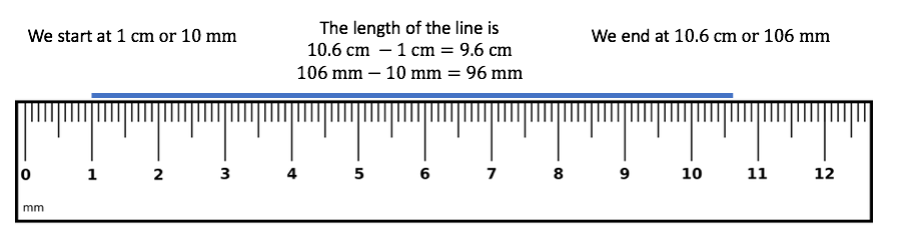Figure 7: Using a ruler to measure a length of $\scriptsize 9.6\ \text{cm}$ or $\scriptsize 96\ \text{mm}$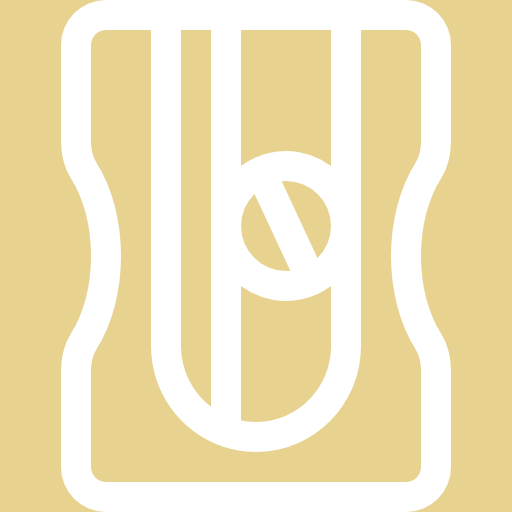### Activity 1.1: Measure length with a ruler

Time required: 10 minutes

What you need:

• an internet connection

What to do:

What did you find?

The lengths of each of the boxes in the first ‘Measuring lengths with a ruler’ activity are as follows:

A: $\scriptsize 120\ \text{mm}$ or $\scriptsize 12\text{ cm}$
B: $\scriptsize 112\ \text{mm}$ or $\scriptsize 11.2\text{ cm}$
C: $\scriptsize 39\ \text{mm}$ or $\scriptsize 3.9\text{ cm}$
D: $\scriptsize 95\ \text{mm}$ or $\scriptsize 9.5\text{ cm}$
E: $\scriptsize 70\ \text{mm}$ or $\scriptsize 7\text{ cm}$

## Measuring angles with a protractor

A protractor is a simple instrument for measuring the size of angles (see Figure 8). Most protractors will measure angles in degrees (the symbol for degrees is $\scriptsize \circ$).

As you can see in Figure 8, a protractor can measure angles between $\scriptsize 0{}^\circ$ and $\scriptsize {{180}^{{}^\circ }}$. To use a protractor, place the middle of the protractor on the vertex of the angle (where the two straight lines, or ‘rays’, meet) such that one of the $\scriptsize 0{}^\circ$ measure lies on one of the lines creating the angle. Then read off the angle created by the other line.

For example, we can see that the size of the angle in Figure 9 is $\scriptsize 77{}^\circ$.Figure 9: Using a protractor to measure an angle of $\scriptsize 77^\circ$

### Note

If you have an internet connection, you should watch the video called “Measuring angles using a protractor”  that demonstrates how to use a protractor.

Measuring angles using a protractor (Duration: 3.25)### Activity 1.2: Measure angles with a protractor

Time required: 10 minutes

What you need:

• an internet connection

What to do:

1. Open the online interactive activity called Measuring angles with a protractor.Here you will find a protractor with which to measure the given angle.
2. Measure the given angle from the line labelled Start in the direction of the arrow. You can click and drag the protractor to move it around. Click and drag on the red dot on the protractor to turn it.
4. Click on the Random button to generate a new random angle to measure. Measure as many angles as you need to become confident.
5. Sometimes the angle you need to measure will be greater than $\scriptsize {{180}^{{}^\circ }}$.
.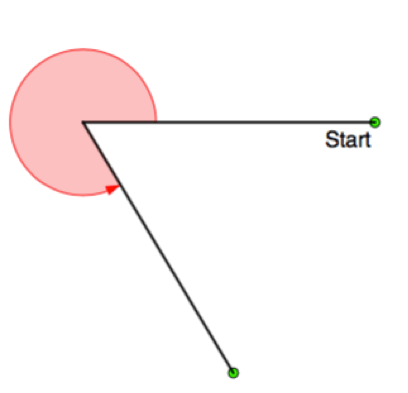.
How will you measure the angle in this case shown here? Here’s a hint. What if you measured the smaller angle created between the two lines that is less than $\scriptsize 180{}^\circ$? What would you subtract this angle from to find the bigger angle that is greater than $\scriptsize 180{}^\circ$? Open the online interactive activity called Measuring angles between 0 and 360 degrees with a protractor.Here you will find a protractor with which to measure angles between $\scriptsize 0{}^\circ$ and $\scriptsize 360{}^\circ$.

What did you find?

Because the online interactive activity generates random angles to measure, it is not possible to give all the answers. But you can check all your answers with the answer box.

Were you able to work out how to measure angles greater than $\scriptsize {{180}^{{}^\circ }}$? To do so, measure the size of the angle smaller than $\scriptsize {{180}^{{}^\circ }}$ created by the two lines and then subtract this angle from $\scriptsize {{360}^{{}^\circ }}$ to find the larger angle. We can do this, because the angles around a point always add up to $\scriptsize {{360}^{{}^\circ }}$.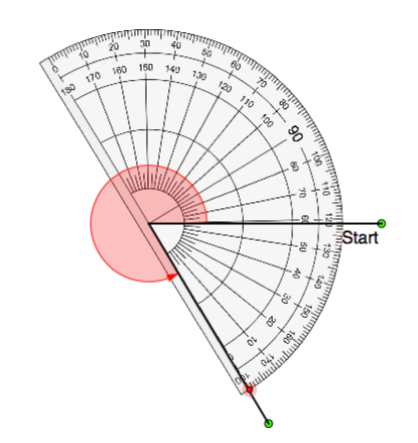### Exercise 1.3

1. Use a ruler to measure the dimensions of the following everyday objects to the nearest $\scriptsize \text{mm}$.
1. The length and width of a piece of A4 paper
2. The height of a $\scriptsize 2\text{ }\ell$ cool drink bottle
3. The height, breadth and depth of a $\scriptsize 1\ \text{kg}$ packet of sugar
2. Use a protractor to measure the following angles to the nearest degree.
1. .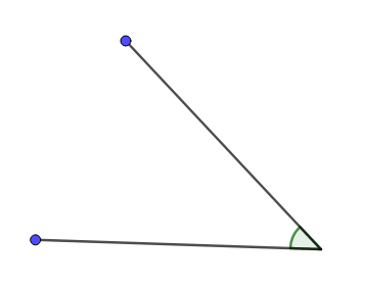2. .3. .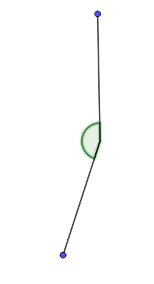4. .The full solutions are at the end of the unit.

## Summary

In this unit you have learnt the following:

• What the SI system of units and measures is.
• How to use a ruler accurately.
• How to use a protractor accurately.

# Unit 1: Assessment

#### Suggested time to complete: 20 minutes

From what you know about these objects, choose the most appropriate answer for Questions 1 to 5.

1. What is the closest to the width of a grape?
1. 15 mm
2. 15 m
3. 15 km
2. What is the closest to the height of a two-story building?
1. 30 m
2. 30 km
3. 30 cm
3. What is the closest to the mass of an apple?
1. 55 mg
2. 55 g
3. 55 kg
4. What would be the best units to use to measure the width of a pen?
1. metres
2. centimetres
3. micrometres
4. millimetres
5. What would be the best units to measure the distance driven by a car
1. metres
2. kilometres
3. centimetres
4. megametres
6. Measure the size of all the angles in this triangle to the nearest degree using a protractor.
.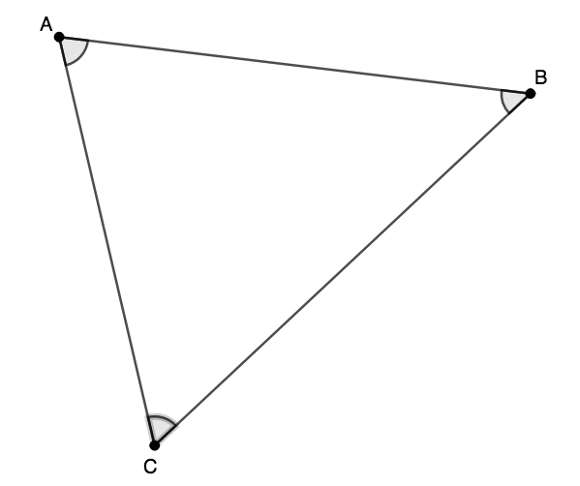7. What is the ratio of the lengths of these two lines? In other words, how many times longer is the long line than the short line?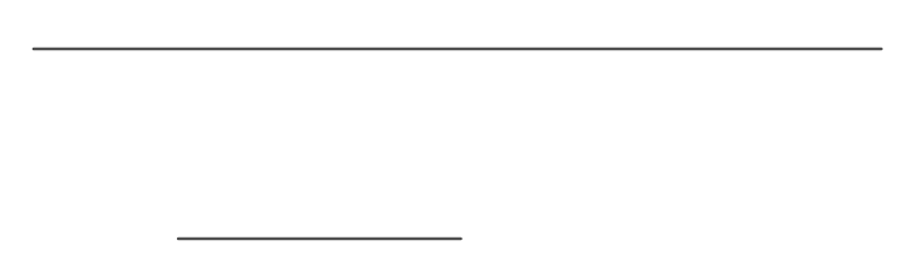8. Jason accelerates to a speed of $\scriptsize 30.56\ \text{km/h}$ in $\scriptsize 9.55\ \text{s}$.
1. To what speed in metres per second does he accelerate?
2. If $\scriptsize a=\displaystyle \frac{s}{t}$ (acceleration equal to speed divided by time), what is Jason’s average rate of acceleration in metres per second per second?

The full solutions are at the end of the unit.

# Unit 1: Solutions

### Exercise 1.1

1. The height of a human adult would be measured in metres.
2. The length of a newborn human would be measured in centimetres (or maybe millimetres).
3. The height of a building would be measured in metres.
4. The mass of a truck would be measured in kilograms or tons where $\scriptsize 1\ \text{ton}=1\ 000\ \text{kg}=1\ \text{Mg}$.
5. The distance from Johannesburg to Cape Town would be measured in kilometres.
6. The mass of a grain of sand would be measured in grams or milligrams.
7. The length of a song would be measured in minutes or seconds.
8. The size of a bacterium would be measured in micrometres or nanometres.
9. The size of an atom would be measured in nanometres.
10. The thickness of a pane of glass would be measured in millimetres.

Back to Exercise 1.1

### Exercise 1.2

1. $\scriptsize \displaystyle \frac{{982\ \text{g}}}{{1\ 000}}=0.982\ \text{kg}$
Therefore, total weight is $\scriptsize 3.67\ \text{kg}+0.982\text{ kg}=4.652\text{ kg}$
Shipping is $\scriptsize \text{R15}$ per kilogram or part. Therefore, shipping will be $\scriptsize \text{R15}\times 5=\text{R}75$.
2. $\scriptsize 3.8\ \text{km}\times 1\ 000=3\ 800\ \text{m}$
Each metre weighs $\scriptsize 280\ \text{g}$. Therefore, all the cable will weigh $\scriptsize 280\ \text{g/m}\times 3800\ \text{m}=1\ 064\ 000\ \text{g}$.
$\scriptsize \displaystyle \frac{{1\ 064\ 000\ \text{g}}}{{1\ 000}}=1\ 064\ \text{kg}$.

Back to Exercise 1.2

### Exercise 1.3

1. .
1. An A4 paper is $\scriptsize 210\ \text{mm}$ wide and $\scriptsize 297\ \text{mm}$ long.
2. The height of a $\scriptsize 2\text{ }\ell$ cool drink bottle is about $\scriptsize 327\ \text{mm}$.
3. A $\scriptsize 1\ \text{kg}$ packet of sugar: height: $\scriptsize 20 \ \text{cm}$, breadth: $\scriptsize 9\ \text{cm}$ , depth: $\scriptsize 5.5\ \text{cm}$
2. .
1. $\scriptsize 45{}^\circ$
2. $\scriptsize 80{}^\circ$
3. $\scriptsize 161{}^\circ$
4. $\scriptsize 241{}^\circ$

Back to Exercise 1.3

### Unit 1: Assessment

1. A)
2. A)
3. B)
4. D)
5. B)
6. Angle A: $\scriptsize 70{}^\circ$, B: $\scriptsize 50{}^\circ$, C: $\scriptsize 60{}^\circ$
7. The longer line is three times as long as the shorter line.
8. .
1. $\scriptsize 30.56\ \text{km/h}\times 1\ 000=30\ 560\ \text{m/h}$
There are $\scriptsize 3\ 600$ seconds in one hour. Therefore $\scriptsize \displaystyle \frac{{30\ 560\ \text{m/h}}}{{3\ 600}}=8.489\ \text{m/s}$
2. $\scriptsize a=\displaystyle \frac{s}{t}=\displaystyle \frac{{8.489\ \text{m/s}}}{{9.55\ \text{s}}}=0.889\ \text{m/s/s}$

Back to Unit 1: Assessment